# Java 8 之 Stream 操作符

## filter

Stream 的 filter() 方法执行过滤操作

Stream<T> filter(Predicate<? super T> predicate);

filter() 方法使用的是 Predicate 的函数接口，用一个图可以很容易理解 Predicate 函数接口所表达的意思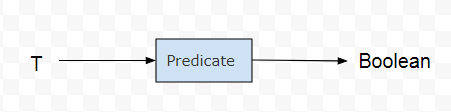Predicate 函数接口用 T 类对象作为参数，返回一个 Boolean 对象用作判断标准。

Stream.of(1, 4, 6).filter(aInteger -> aInteger > 3);

Lambda 表达式 aInteger -> aInteger > 3 的意思是说，取 Stream 中的元素 aInteger，给出判断标准是 aInteger > 3，然后用 filter() 方法进行过滤。

## map

Stream 的 map() 方法，简单的说就是把一种类型的流，转换为另外一种类型的流

<R> Stream<R> map(Function<? super T, ? extends R> mapper);

map() 方法使用的是 Function 函数接口，还是用一个图来理解 Function 函数接口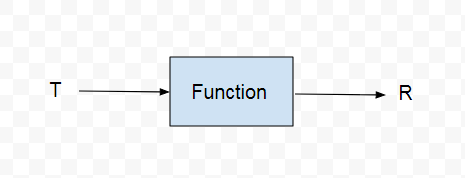Stream<Integer> integerStream = Stream.of(1, 2, 3);
Stream<String> stringStream = integerStream.map(String::valueOf);

## mapToInt, mapToLong, mapToDouble

IntStream mapToInt(ToIntFunction<? super T> mapper);
LongStream mapToLong(ToLongFunction<? super T> mapper);
DoubleStream mapToDouble(ToDoubleFunction<? super T> mapper);

Java 的基本类型，都有对应的包装类型，例如 int 对应 Integer。然而对于泛型，只能操作包装类型，例如 Integer。然而包装类型对象相比于基本类型内存开销要大的。

Java 有 8 中基本类型，然而 Java 8 中却只有对应的三种基本 Stream。

        Stream<Integer> integerStream = Stream.of(1, 2, 3);
IntStream intStream = integerStream.mapToInt(integer -> integer);

        OptionalInt min = Stream.of(1, 2, 3)
.mapToInt(integer -> integer)
.min();

### Optional

Optional 是一个容器，它可以包含或者不包含一个值，如果值存在，isPresent() 就返回 true，get() 就返回包含的值。Optional 就是对一个值进行一次包装，好像就是为了对付空指针异常设计的，使用方法如下。

        if (optionalInt.isPresent()) {
int min = optionalInt.getAsInt();
}

orElse(T other) 会返回一个默认值，如果值不存在，更有甚者 orElseGet(Supplier< ? extends T> other)，当值不存在的时候，执行一个操作来返回一个默认值。
ifPresent(Consumer

        Stream.of(1, 2, 3)
.mapToInt(integer -> integer)
.min()
.ifPresent(System.out::println);    

### IntSummaryStatistics

1. IntStream 的 min() 返回值是 OptionalInt, 需要检查返回值是否为 null，而 IntSummaryStatistics 的 getMin() 方法返回的是基本类型 Int，自动过滤了 null 值，因此后者更快。
2. 上篇文章对 Stream 讲解的时候说到，Stream 的一次性特性，
一个 Stream 只能被一个操作符操作，而且只能操作一次。 如果不把 IntStream 转换为 IntSummaryStatistics, 如果想既求出最大值也求出最小值，那就不行了。因此用统计的数据的角度看，后者也更胜一筹，个人觉得这也是主要原因吧。

        IntSummaryStatistics intSummaryStatistics = Stream.of(1, 2, 3)
.mapToInt(integer -> integer)
.summaryStatistics();
intSummaryStatistics.accept(888);
int max = intSummaryStatistics.getMax();

max 的值获取到的为 888.

combine(IntSummaryStatistics other) 方法，用来合并另外一个 IntSummaryStatistics

## flatMap

Stream 的 flatMap() 方法，从返回值看，就是把 Stream< T> 转换为 Stream< R>，但是 flatMap() 调用的函数接口 Function ，是把类型 T 转换为 Stream < R> ，这点是需要注意的。

        Person user1 = new Person().setName("user1").setAge(18).setWhere("GuangDong", "ShenZhen");
Person user2 = new Person().setName("user2").setAge(28).setWhere("HuBei", "WuHan");
Stream<Person> userStream = Stream.of(user1, user2);
Stream<String> stringStream = userStream
.flatMap(Person::getFromWhere);

public Stream<String> getFromWhere() {
return fromWhere.stream();
}               

Lambda 表达式 Person::getFromWhere 其实是把 Stream< Person> 转换为了 Stream< Stream< String>>，而 flatMap() 最终就把 Stream< Stream< String>> 转换为 Stream< String>。

## flatMapToInt, flatMapToLong, flatMapToDouble

IntStream flatMapToInt(Function<? super T, ? extends IntStream> mapper);

        IntStream intStream = Stream.of(user1, user2)
.flatMapToInt(person -> IntStream.of(person.getAge()));

## forEach, forEachOrdered

void forEach(Consumer<? super T> action);
void forEachOrdered(Consumer<? super T> action);

1. forEach()：对于并行的流，并不能保证流中元素的顺序，如果保证了顺序就会牺牲掉并行所带来的好处。对于任意一个元素，它的 action 可能会在任意时间，任意线程中执行，这是由库选择的。如果 action 要访问一个共享状态，这个方法需要提供了同步机制。
2. forEachOrdered()：按照 Stream 中元素的顺序执行 action，也就是说同一时间只有一个 action 在进行，但是这个 action 可能在任意线程中执行，这也是由库选择的。
        Stream.of(user1, user2)
.parallel()
.forEach(person -> {
System.out.println(person);
});

System.out: main
System.out: Person{name='user2', age=28, fromWhere='null'}
System.out: ForkJoinPool.commonPool-worker-1
System.out: Person{name='user1', age=18, fromWhere='null'}


## distinct

Stream<T> distinct();

        Stream.of("a", "b", "a", "c")
.distinct()
.forEach(System.out::print); 

System.out: a
System.out: b
System.out: c

## sorted

Stream<T> sorted();
Stream<T> sorted(Comparator<? super T> comparator);

2个方法都是对 Stream 中的元素进行排序，sorted() 使用的是自然排序，而 sorted(comparator) 需要给定一个排序标准。

        Stream.of(user1, user2, user3)
.sorted((person1, person2) -> person2.getAge() - person1.getAge())
.forEach(person -> System.out.println(person.getName()));

Java 8 中给函数接口 Comparator 创建一个 default 方法 comparing 方法

    static default <T, U extends Comparable<? super U>> Comparator<T> comparing(Function<? super T, ? extends U> keyExtractor) {
// ...
}

        Stream.of(user1, user2, user3)
.sorted(Comparator.comparing(Person::getAge))
.forEach(person -> System.out.println(person.getName()));

Comparator.comparing(Person::getAge) 这个 Lambda 表达式可以这样理解，它创建了一个标准，这个标准就是来比较 Person 的 Age，sorted() 方法用这个标准来排序。

## peek

Stream<T> peek(Consumer<? super T> action);

peek() 方法在 Collection 类中是获取队首的元素( 但不移除 )，而在 Stream 中，也获取了从 Up Stream 中元素，但是也额外在这个元素上用 action() 方法做了一些操作，

        Stream<Person> peek = Stream.of(user1, user2, user3)
.peek(System.out::println);

## limit

Stream<T> limit(long maxSize);

limit() 方法从返回值看，这是一个过渡操作符，并且限制 Up Stream 发送元素的个数不大于 maxSize。

        long count = Stream.generate(() -> new Random().nextBoolean())
.limit(5)
.peek(System.out::println)
.count();

## skip

Stream<T> skip(long n);

skip() 方法，很简单，跳过前 n 个元素，这个方法也是一个过渡方法。

skip() 与 limit() 一样，在遇到并行且有序的 Stream 的时候，是有开销的，Stream 越大开销越大。

## toArray

Object[] toArray();
<A> A[] toArray(IntFunction<A[]> generator);

        Person[] persons = Stream.of(user1, user2, user3)
.toArray(new IntFunction<Person[]>() {
@Override
public Person[] apply(int i) {
return new Person[i];
}
});

IntFunction 函数接口返回了一个大小为 i 的 Person 数组，这个 i 表示的是 Stream 中元素的个数。

        Person[] persons = Stream.of(user1, user2, user3)
.toArray(Person[]::new);

## match

boolean anyMatch(Predicate<? super T> predicate);
boolean allMatch(Predicate<? super T> predicate);
boolean noneMatch(Predicate<? super T> predicate);

anyMatch() ：如果 Stream 是空 (empty) 的，返回 false，并且 predicate 不会执行。

allMatch(), noneMatch：如果 Stream 是空 (empty) 的，返回 true，并且 predicate 不会执行。

boolean b = Stream.empty().noneMatch(o -> false); // true

## find

Optional<T> findFirst();
Optional<T> findAny();

findFirst() 获取 Steam 中第一个元素，如果 Stream 是无序的，这第一个元素就不确定。
findAny() 就更随意，获取任意一个元素。

        Stream.of(user1, user2, user3)
.findFirst()
.ifPresent(System.out::println); // user1

## min, max

Optional<T> min(Comparator<? super T> comparator);
Optional<T> max(Comparator<? super T> comparator);

Optional<Person> maxPerson = Stream.of(user1, user2, user3).max(Comparator.comparing(Person::getAge));

## count

long count(); 

## reduce

T reduce(T identity, BinaryOperator<T> accumulator);

Optional<T> reduce(BinaryOperator<T> accumulator);

<U> U reduce(U identity,
BiFunction<U, ? super T, U> accumulator,
BinaryOperator<U> combiner);

    private int sum(List<Integer> integers) {
int result = 0;
for (Integer integer : integers) {
result = result + integer;
}
return result;
}

    T result = identity;
for (T element : this stream)
result = accumulator.apply(result, element)
return result;

T reduce(T identity, BinaryOperator<T> accumulator);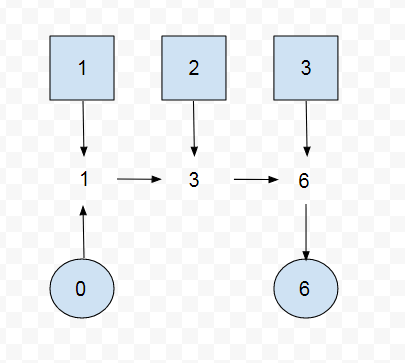Reduction 模式也可以不要初始值，那么就对应 reduce 方法的第二个方法

Optional<T> reduce(BinaryOperator<T> accumulator);

        Optional<Integer> sum = Stream.of(user1, user2, user3)
.map(Person::getAge)
.reduce((age1, age2) -> age1 + age2);

ok, 现在看看三个参数的 reduce() 方法

<U> U reduce(U identity,
BiFunction<U, ? super T, U> accumulator,
BinaryOperator<U> combiner);

        Integer sum = Stream.of(1, 2, 3)
.reduce(100,
(result, integer) -> {
System.out.println("in accumulator, result = "
+ result + ", integer = " + integer);
return result + integer;
},
(result1, result2) -> {
System.out.println("in combiner, result1 = "
+ result1 + ", result2 = " + result2);
return result1 + result2;
});
System.out.println(sum);

Stream.of(1,2,3) 是顺序执行的 Stream，虽然是为了求 1+2+3 的和，但是我这里为了更好的看出执行过程，把初始值设置为 100，这就等于是 100 + 1 + 2 + 3 = 106。 执行这个程序后会有如下 Log

System.out: in accumulator, result = 100, integer = 1
System.out: in accumulator, result = 101, integer = 2
System.out: in accumulator, result = 103, integer = 3
System.out: 106

Log 中 result 代表累加的结果，初始的时候，result 等于设置的初始值 100， 然后累加第一个元素 1， result 就变为 101， 之后累加第二个元素 2， result 变为 103，最后累加第三个元素 3， result 的值其实已经变为 106, 也就是最终的返回值。

        Integer sum = Stream.of(1, 2, 3)
.parallel() // 改为并行流
.reduce(100,
(result, integer) -> {
System.out.println("in accumulator, result = "
+ result + ", integer = " + integer);
return result + integer;
},
(result1, result2) -> {
System.out.println("in combiner, result1 = "
+ result1 + ", result2 = " + result2);
return result1 + result2;
});
System.out.println(sum);

System.out: in accumulator, result = 100, integer = 2
System.out: in accumulator, result = 100, integer = 3
System.out: in accumulator, result = 100, integer = 1
System.out: in combiner, result1 = 102, result2 = 103
System.out: in combiner, result1 = 101, result2 = 205
System.out: 306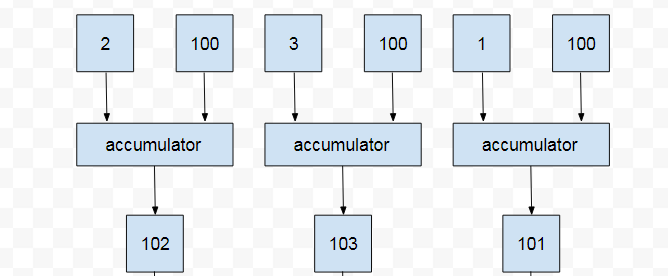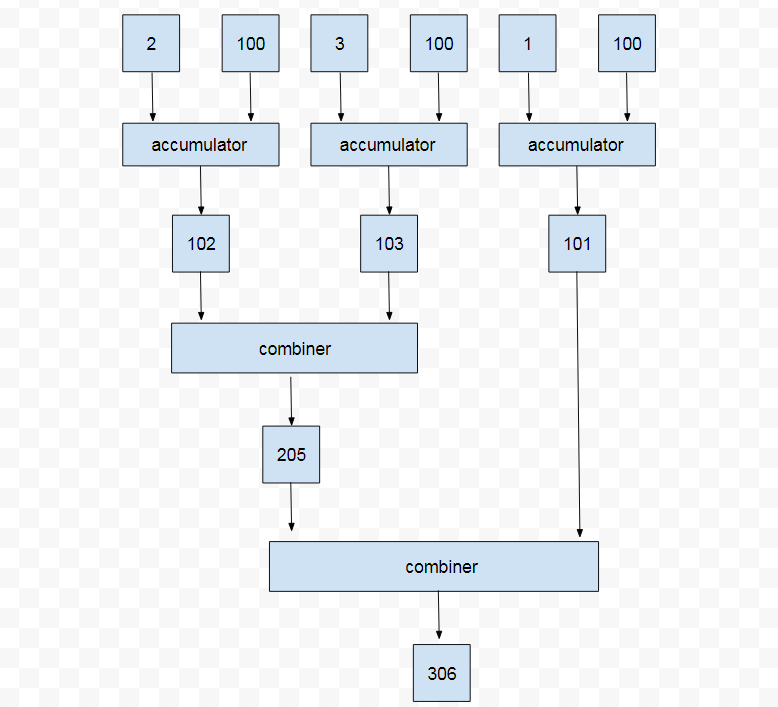combiner.apply(u, accumulator.apply(identity, t)) == accumulator.apply(u, t)

## collect

    <R, A> R collect(Collector<? super T, A, R> collector);

<R> R collect(Supplier<R> supplier,
BiConsumer<R, ? super T> accumulator,
BiConsumer<R, R> combiner);

### 转换成集合

<R, A> R collect(Collector<? super T, A, R> collector);

    public static <T>
Collector<T, ?, List<T>> toList() {
// ...
}

public static <T>
Collector<T, ?, Set<T>> toSet() {
// ...
}

public static <T, C extends Collection<T>>
Collector<T, ?, C> toCollection(Supplier<C> collectionFactory) {
// ...
}

List<Integer> list = Stream.of(1, 2, 3).collect(Collectors.toList());

Set<Integer> set = Stream.of(1, 2, 3).collect(Collectors.toSet());

LinkedList<Integer> queue = Stream.of(1, 2, 3).collect(Collectors.toCollection(LinkedList::new));

### 转化为 Map

    public static <T, K, U>
Collector<T, ?, Map<K,U>> toMap(Function<? super T, ? extends K> keyMapper,
Function<? super T, ? extends U> valueMapper) {
// ...
}

public static <T, K, U>
Collector<T, ?, Map<K,U>> toMap(Function<? super T, ? extends K> keyMapper,
Function<? super T, ? extends U> valueMapper,
BinaryOperator<U> mergeFunction) {
// ...
}

public static <T, K, U, M extends Map<K, U>>
Collector<T, ?, M> toMap(Function<? super T, ? extends K> keyMapper,
Function<? super T, ? extends U> valueMapper,
BinaryOperator<U> mergeFunction,
Supplier<M> mapSupplier) {
// ...
}


        Map<String, Integer> nameAges = Stream.of(user1, user2, user3)
.collect(Collectors.toMap(Person::getName, Person::getAge));

Map 的 key 值是不能重复的，而如果上面例子中，Person 的 name 有重复的，就会报异常

java.lang.IllegalStateException: Duplicate key

        Map<String, String> map = Stream.of(user1, user2, user3)
.collect(Collectors.toMap(Person::getName,
Person::getPhoneNumber,
(number1, number2) -> number1 + "," + number2));

        HashMap<String, String> treeMap = Stream.of(user1, user2, user3)
.collect(Collectors.toMap(Person::getName,
Person::getPhoneNumber,
(number1, number2) -> number1 + "," + number2,
HashMap::new));

Collectors.toConcurrentMap() 的参数与 Collectors.toMap() 一样， 只是toConcurrentMap() 返回的是一个并发的 Collector 对象。

Collectors 还有一个名字与 map 有关的方法，叫做 mapping

    public static <T, U, A, R>
Collector<T, ?, R> mapping(Function<? super T, ? extends U> mapper,
Collector<? super U, A, R> downstream) {
// ...
}

        List<String> list = Stream.of(user1, user2, user3)
.collect(Collectors.mapping(Person::getName, Collectors.toList()));

        long count = Stream.of(user1, user2, user3)
.collect(Collectors.mapping(Person::getName, Collectors.counting()));

        List<String> names = Stream.of(user1, user2, user3)
.map(Person::getName)
.collect(Collectors.toList());

### 转换成一个值

Collectors 还有和 Steram 一样求单一值的方法，例如, 最大/小值(maxBy()/minBy()), 计数(counting()),

Optional<Person> min = Stream.of(user1, user2).collect(Collectors.minBy(Comparator.comparing(Person::getAge)));
Double average = Stream.of(user1, user2).collect(Collectors.averagingInt(Person::getAge));

### 数据分块

Collectors 的 partitioningBy() 可以将 Stream 分块

    public static <T, K> Collector<T, ?, Map<K, List<T>>>
groupingBy(Function<? super T, ? extends K> classifier) {
// ...
}

public static <T, K, A, D>
Collector<T, ?, Map<K, D>> groupingBy(Function<? super T, ? extends K> classifier,
Collector<? super T, A, D> downstream) {
// ...
}

        Map<Boolean, List<Person>> map = Stream.of(user1, user2, user3)
.collect(Collectors.partitioningBy(person -> person.getAge() > 20));

        Map<Boolean, Set<String>> map = Stream.of(user1, user2, user3)
.collect(Collectors.partitioningBy(person -> person.getAge() > 20,
Collectors.mapping(Person::getName, Collectors.toSet())));

### 数据分组

    public static <T, K> Collector<T, ?, Map<K, List<T>>>
groupingBy(Function<? super T, ? extends K> classifier) {
// ...
}

public static <T, K, A, D>
Collector<T, ?, Map<K, D>> groupingBy(Function<? super T, ? extends K> classifier,
Collector<? super T, A, D> downstream) {
// ...
}

public static <T, K, D, A, M extends Map<K, D>>
Collector<T, ?, M> groupingBy(Function<? super T, ? extends K> classifier,
Supplier<M> mapFactory,
Collector<? super T, A, D> downstream) {
//...
}



        Map<Integer, List<Person>> group = Stream.of(user1, user2)
.collect(Collectors.groupingBy(Person::getAge));

        Map<Integer, Long> ageCountParis = Stream.of(user1, user2, user3)
.collect(Collectors.groupingBy(Person::getAge, Collectors.counting()));

        HashMap<Integer, Set<Person>> collect = Stream.of(user1, user2, user3)
.collect(Collectors.groupingBy(Person::getAge, HashMap::new, Collectors.toSet()));

### 字符串链接

    public static Collector<CharSequence, ?, String> joining() {
// ...
}

public static Collector<CharSequence, ?, String> joining(CharSequence delimiter) {
// ...
}

public static Collector<CharSequence, ?, String> joining(CharSequence delimiter,
CharSequence prefix,
CharSequence suffix) {
// ...
}       

        String str1 = Stream.of("a", "b", "c", "d").collect(Collectors.joining()); // abcd
String str2 = Stream.of("a", "b", "c", "d").collect(Collectors.joining(",")); // a,b,c,d
String str3 = Stream.of("a", "b", "c", "d").collect(Collectors.joining(",")); // [a,b,c,d]

### reducing

    public static <T> Collector<T, ?, T>
reducing(T identity, BinaryOperator<T> op) {}

public static <T> Collector<T, ?, Optional<T>>
reducing(BinaryOperator<T> op) {}

public static <T, U>
Collector<T, ?, U> reducing(U identity,
Function<? super T, ? extends U> mapper,
BinaryOperator<U> op) {}    

Colletors 的一个和两个参数的 reducing() 与 Stream 的 reduce() 方法一样，那么看下三个参数的方法。

        Integer sumOfAge = Stream.of(user1, user2, user3)
.collect(Collectors.reducing(0, Person::getAge, Integer::sum));

Collectors.reducing() 和 Collectors.mapping() 方法一样，是用来组合其他 Collector 的，尤其是在组合 Collectors.groupingBy() 和 Collectors.partitioningBy()

        Map<Person.Sex, Integer> collect = Stream.of(user1, user2, user3)
.collect(Collectors.groupingBy(Person::getSex,
Collectors.reducing(0, Person::getAge, Integer::sum)));

### 自定义 Collector

Stream 的三个参数的 Collect() 方法就是使用自定义的 Collector

    <R> R collect(Supplier<R> supplier,
BiConsumer<R, ? super T> accumulator,
BiConsumer<R, R> combiner);

        Supplier supplier = new Supplier<ArrayList>() {
@Override
public ArrayList get() {
return new ArrayList();
}
};

        BiConsumer accumulator = new BiConsumer<ArrayList, Person>() {
@Override
public void accept(ArrayList arrayList, Person person) {
}
};

        BiConsumer combiner = new BiConsumer<ArrayList, ArrayList>() {
@Override
public void accept(ArrayList arrayList, ArrayList arrayList2) {
}
};

        ArrayList<Person> persons = Stream.of(user1, user2, user3)
.collect(ArrayList::new, ArrayList::add, ArrayList::addAll);©️2019 CSDN 皮肤主题: 像素格子 设计师: CSDN官方博客